# SIMP Method for Topology Optimization

Topology optimization is the most common type of structural optimization. It is used in the initial phase of the design to predict the optimal material distribution within a given initial design space of a structure and considers functional specifications and manufacturing constraints.

The most popular mathematical method for topology optimization is the Solid Isotropic Material with Penalization method (SIMP). Bendsoe and Kikuchi (1988) and Rozvany and Zhou (1992). initially proposed the SIMP method. The SIMP method predicts an optimal material distribution within a given design space, for given load cases, boundary conditions, manufacturing constraints, and performance requirements.

According to Bendsoe (1989): "shape optimization in its most general setting should consist of a determination for every point in space whether there is material in that point or not." The traditional approach to topology optimization is the discretization of a domain into a grid of finite elements called isotropic solid microstructures. Each element is either filled with material for regions that require material, or emptied of material for regions where you can remove material (representing voids). The density distribution of material within a design domain, ρ, is discrete, and each element is assigned a binary value:
• ρ(e) = 1 where material is required (black)
• ρ(e) = 0 where material is removed (white)

For example, the image shows an optimized material layout of a loaded beam. The solid elements with densities ρ(e) =1 are black, whereas the void elements with ρ(e) = 0 are removed.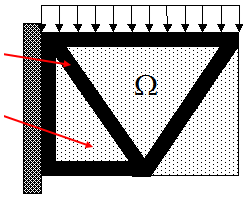The introduction of a continuous relative density distribution function avoids the binary, on-off nature of the problem. For each element, the assigned relative density can vary between a minimum value ρmin and 1, which allows the assignment of intermediate densities for elements (characterized as porous elements):

ρmin is the minimum allowable relative density value for empty elements that are greater than zero. This density value ensures the numerical stability of the finite element analysis.

Since the material relative density can vary continuously, the material Young modulus at each element can also vary continuously. For each element e the relation between the material relative density factor ρe and the Young modulus of elasticity of the assigned isotropic material model Ε0 is computed by the power law: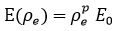The penalty factor p diminishes the contribution of elements with intermediate densities (gray elements) to the total stiffness. The penalty factor steers the optimization solution to elements that are either solid black (ρe = 1) or void white (ρe= ρmin). Numerical experiments indicate that a penalty factor value of p = 3 is suitable.

A reduction of an element’s material elastic modulus leads to a reduction of element stiffness. According to the SIMP method, the global stiffness is modulated according to: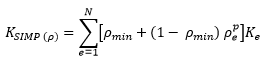whereis the element stiffness matrix, ρmin is the minimum relative density, ρe is the element relative density, p is the penalty factor, and N is the number of elements in the design domain.

For example, for an element with an assigned relative density ρe = 0.5, penalty factor = 3, and ρmin = 0.001, the global stiffness matrix is scaled by a factor of (0.001 + (1 -0.001)* 0.5 ^3) = 0.12587.

## Objective Function: Maximizing Stiffness

A popular optimization objective is to maximize the overall stiffness of a structure, or minimize its compliance under a given amount of mass removal.

Compliance is a measure of the overall flexibility or softness of a structure, and is the reciprocal of stiffness. The global compliance is equal to the sum of the element elastic or strain energies. Minimizing the global compliance, C, is equivalent to maximizing the global stiffness. The optimization algorithm, through an iterative process, seeks to resolve the element densities (which are the optimization design variables) that minimize the global compliance of the structure.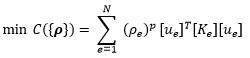[ue] is the nodal displacement vector of element e, [Ke] is the stiffness of element e, and vector {ρ} contains the elements' relative densities ρe.

During each optimization iteration, the target mass constraint, the global force-stiffness equilibrium, and the required functional constraints must be satisfied:ve is the element volume, and Mtarget is the target mass of the optimization.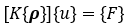[K{ρ}] is the global stiffness matrix modulated by the vector of relative densities, {u} is the displacement vector, and {F} is the external force vector.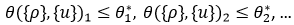The formula above contains design response constraints such as limits on stresses, displacements, eigenfrequencies, etc.

## Sensitivity Analysis

During each iteration, the optimization algorithm performs a sensitivity analysis to evaluate the impact the variation of material densities has on the objective function to maximize stiffness.

Mathematically, the sensitivity analysis is expressed as the derivative of the objective function with respect to the material densities: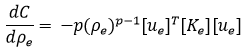During a sensitivity analysis, elements weighted with low material density factors eventually lose their structural importance and are eliminated during further iterations.

If you calculate the sensitivity for each element independently and don't consider the connectivity between elements, this can lead to material discontinuity and to volumes becoming unconnected to the main geometry. This is known as checkerboard effect. To reduce the checkerboard effect, a filtering scheme applies an element influence radius and averages the sensitivities of each element inside its influence region.

The optimization iterations continue until the variations of the objective function converge and iterations reach their converge criteria.